Paracompactness criteria

The following statements are equivalent for an arbitrary completely-regular Hausdorff space(cf. Completely-regular space; Hausdorff space).

1)is paracompact.

2) Each open covering ofcan be refined to a locally finite open covering.

3) Each open covering ofcan be refined to a-locally finite open covering, i.e. an open covering decomposing into a countable collection of locally finite families of sets in.

4) Each open covering ofcan be refined to a locally finite covering (about the structure of the elements of which nothing is assumed).

5) For any open coveringofthere exists an open covering which is a star refinement of.

6) Each open covering ofcan be refined to a conservative covering.

7) For any open coveringofthere exists a countable collection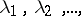of open coverings of this space such that for each pointand for each of its neighbourhoods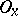there exist a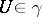and an integersatisfying the condition: Each element of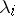intersectingis contained in(i.e. each star of the setrelative tolies in).

8) For any open coveringofthere exists a continuous mapping of the spaceinto some metric spacesubject to the condition: At each point ofthere exists a neighbourhood whose inverse image is contained in an element of.

9) The spaceis collectionwise normal and weakly paracompact.

Additional equivalent statements are:

10) The product ofand any compact Hausdorff space is normal (cf. Normal space).

11)is normal.

12) Every lower semi-continuous multi-valued mapping fromto a Banach space contains a continuous single-valued mapping.

13)admits a uniformity for which the hyperspace of closed sets is complete.

Such a mapping as is posited in 8) is said to realize the covering.

Weakly paracompact spaces are also called metacompact. They are the spaces every open covering of which has a point-finite open refinement.

A family of sets, in particular a covering, is called a conservative family of sets if for every subfamily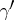of,. Heredenotes the closure of.